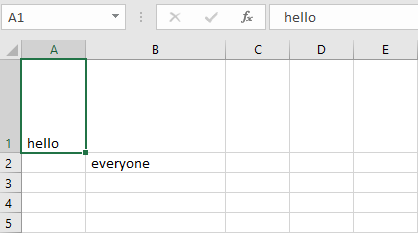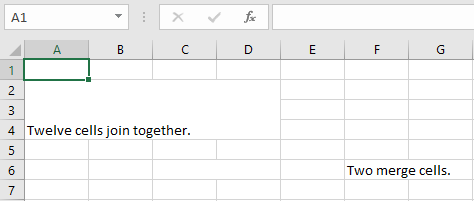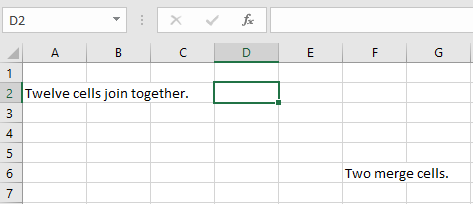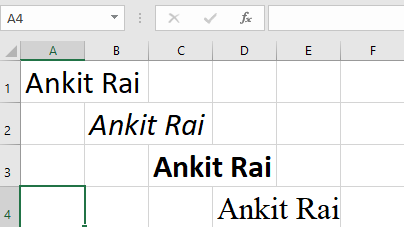GeeksforGeeks App
Open AppBrowser
Continue

# Python | Adjusting rows and columns of an excel file using openpyxl module

Prerequisites : Excel file using openpyxl writing | reading

#### Set the height and width of the cells:

Worksheet objects have `row_dimensions` and `column_dimensions` attributes that control row heights and column widths. A sheet’s `row_dimensions` and `column_dimensions `are dictionary-like values; row_dimensions contains RowDimension objects and column_dimensions contains ColumnDimension objects. In row_dimensions, one can access one of the objects using the number of the row (in this case, 1 or 2). In column_dimensions, one can access one of the objects using the letter of the column (in this case, A or B).

Code #1 : Program to set the dimensions of the cells.

 `# import openpyxl module``import` `openpyxl`` ` `# Call a Workbook() function of openpyxl ``# to create a new blank Workbook object``wb ``=` `openpyxl.Workbook()`` ` `# Get workbook active sheet  ``# from the active attribute. ``sheet ``=` `wb.active`` ` `# writing to the specified cell``sheet.cell(row ``=` `1``, column ``=` `1``).value ``=` `' hello '`` ` `sheet.cell(row ``=` `2``, column ``=` `2``).value ``=` `' everyone '`` ` `# set the height of the row``sheet.row_dimensions[``1``].height ``=` `70`` ` `# set the width of the column``sheet.column_dimensions[``'B'``].width ``=` `20`` ` `# save the file``wb.save(``'dimension.xlsx'``)`

Output:#### Merging the cells:

A rectangular area of cells can be merged into a single cell with the merge_cells() sheet method. The argument to `merge_cells()` is a single string of the top-left and bottom-right cells of the rectangular area to be merged.

Code #2 : Program to merge the cells.

 `import` `openpyxl``wb ``=` `openpyxl.Workbook()``sheet ``=` `wb.active`` ` `# merge cell from A2 to D4 i.e.``# A2, B2, C2, D2, A3, B3, C3, D3, A4, B4, C4 and D4 .``# A2:D4' merges 12 cells into a single cell. ``sheet.merge_cells(``'A2:D4'``)`` ` `sheet.cell(row ``=` `2``, column ``=` `1``).value ``=` `'Twelve cells join together.'`` ` `# merge cell C6 and D6``sheet.merge_cells(``'C6:D6'``)`` ` `sheet.cell(row ``=` `6``, column ``=` `6``).value ``=` `'Two merge cells.'`` ` `wb.save(``'merge.xlsx'``)`

Output:#### Unmerging the cells:

To unmerge cells, call the unmerge_cells() sheet method.

Code #3 : Program to unmerge the cells.

 `import` `openpyxl``wb ``=` `openpyxl.load_workbook(``'merge.xlsx'``)``sheet ``=` `wb.active`` ` `# unmerge the cells``sheet.unmerge_cells(``'A2:D4'``)`` ` `sheet.unmerge_cells(``'C6:D6'``)`` ` `wb.save(``'merge.xlsx'``)`

Output:#### Setting the font styles of the cells:

To customize font styles in cells, important, import the Font() function from the openpyxl.styles module.

Code #4 : Program to set the font of the text.

 `import` `openpyxl`` ` `# import Font function from openpyxl``from` `openpyxl.styles ``import` `Font`` ` `wb ``=` `openpyxl.Workbook()``sheet ``=` `wb.active`` ` `sheet.cell(row ``=` `1``, column ``=` `1``).value ``=` `"Ankit Rai"`` ` `# set the size of the cell to 24``sheet.cell(row ``=` `1``, column ``=` `1``).font ``=` `Font(size ``=` `24` `)`` ` `sheet.cell(row ``=` `2``, column ``=` `2``).value ``=` `"Ankit Rai"`` ` `# set the font style to italic``sheet.cell(row ``=` `2``, column ``=` `2``).font ``=` `Font(size ``=` `24``, italic ``=` `True``)`` ` `sheet.cell(row ``=` `3``, column ``=` `3``).value ``=` `"Ankit Rai"`` ` `# set the font style to bold``sheet.cell(row ``=` `3``, column ``=` `3``).font ``=` `Font(size ``=` `24``, bold ``=` `True``)`` ` `sheet.cell(row ``=` `4``, column ``=` `4``).value ``=` `"Ankit Rai"`` ` `# set the font name to 'Times New Roman'``sheet.cell(row ``=` `4``, column ``=` `4``).font ``=` `Font(size ``=` `24``, name ``=` `'Times New Roman'``)`` ` `wb.save(``'styles.xlsx'``)`

Output:My Personal Notes arrow_drop_up